### 9.2.4.2 Parameter estimation

Another important application of the decision-making framework of this section is parameter estimation [89,268]. In this case, nature selects a parameter,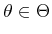, and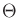represents a parameter space. Through one or more independent trials, some observations are obtained. Each observation should ideally be a direct measurement of, but imperfections in the measurement process distort the observation. Usually,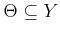, and in many cases,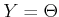. The robot action is to guess the parameter that was chosen by nature. Hence,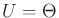. In most applications, all of the spaces are continuous subsets of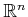. The cost function is designed to increase as the error,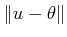, becomes larger.

Example 9..12 (Parameter Estimation)   Suppose that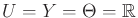. Nature therefore chooses a real-valued parameter, which is estimated. The cost of making a mistake is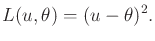(9.35)

Suppose that a Bayesian approach is taken. The prior probability density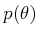is given as uniform over an interval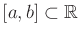. An observation is received, but it is noisy. The noise can be modeled as a second action of nature, as described in Section 9.2.3. This leads to a density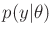. Suppose that the noise is modeled with a Gaussian, which results in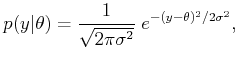(9.36)

in which the mean is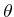and the standard deviation is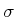.

The optimal parameter estimate based on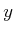is obtained by selecting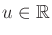to minimize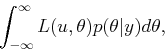(9.37)

in which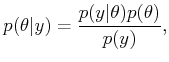(9.38)

by Bayes' rule. The term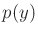does not depend on, and it can therefore be ignored in the optimization. Using the prior density,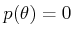outside of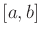; hence, the domain of integration can be restricted to. The value of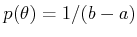is also a constant that can be ignored in the optimization. Using (9.36), this means thatis selected to optimize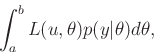(9.39)

which can be expressed in terms of the standard error function,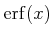(the integral from 0 to a constant, of a Gaussian density over an interval).

If a sequence,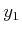,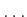,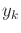, of independent observations is obtained, then (9.39) is replaced by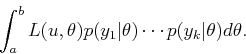(9.40)Steven M LaValle 2020-08-14